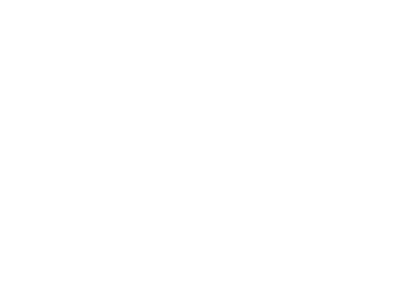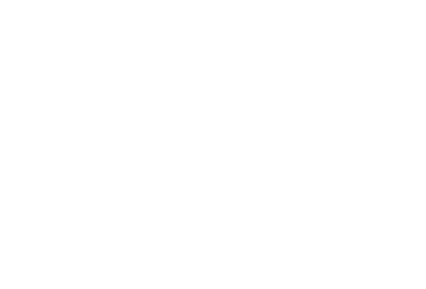# Profit Maximization Methods in Managerial Economics

The profit  maximization  theory states that firms (companies or corporations) will  establish factories where they see the potential to achieve the highest total profit. The  company will select a location based upon comparative advantage (where the product can  be produced the cheapest). The theory draws from the characteristics of the location site,  land price,  labor  costs, transportation costs and access, environmental restrictions, worker  unions, population etc. The company will then elect the best location for the factory to  maximize  profits. This is anathema to the idea of social responsibility because firms will  place their factory to achieve profit  maximization.  They are nonchalant to environment  conservation, fair wage policies and exploit the country. The only objective is to earn more  profits. In economics, profit  maximization  is the process by which a firm determines the  price and output level that returns the greatest profit. There are several  approaches to profit maximization.

## 1. Total Cost-Total Revenue Method

To obtain the profit  maximizing  output quantity, we start by  recognizing  that profit is  equal to total revenue (TR) minus total cost (TC). Given a table of costs and revenues at each  quantity, we can either compute equations or plot the data directly on a graph. Finding the  profit-maximizing  output is as simple as finding the output at which profit reaches its  maximum. That is represented by output Q in the diagram.There are two graphical ways of determining that Q is optimal. Firstly, we see that  the profit curve is at its maximum at this point (A). Secondly, we see that at the point (B)  that the tangent on the total cost curve (TC) is parallel to the total revenue curve (TR), the  surplus of revenue net of costs (BC) is the greatest.  Because total revenue minus total costs  is equal to profit, the line segment CB is equal in length to the line segment AQ.

Computing the price, at which the product  should be sold, requires knowledge of the  firm’s demand curve. Optimum price to sell the product is  the price at which quantity  demanded equals profit-maximizing  output.

## 2. Marginal Cost-Marginal Revenue MethodAn alternative argument says that for each unit sold, marginal profit (MÏ€) equals  marginal revenue (MR) minus marginal cost (MC). Then, if marginal revenue is greater than  marginal cost, marginal profit is positive, and if marginal revenue is less than marginal cost,  marginal profit is negative. When marginal revenue equals marginal cost, marginal profit is  zero. Since total profit increases when marginal profit is positive and total profit decreases  when marginal profit is negative, it must reach a maximum  where marginal profit is zero or marginal cost equals marginal revenue. If there are two points where this occurs,  maximum profit is achieved where the producer was collected positive profit up until the  intersection of MR and MC (where zero profit is collected), but would not continue to after,  as opposed to vice versa, which represents a profit minimum. In calculus terms, the correct  intersection of MC and MR will occur when:

dMR/dQ < dMC/dQ

The intersection of MR and MC is shown in the next diagram as point A. If the  industry is perfectly competitive (as is assumed in the diagram), the firm faces a demand  curve (D) that is identical to its Marginal revenue curve (MR), and this is a horizontal line at a  price determined by industry supply and demand. Average total costs are represented by  curve ATC. Total economic profit are represented by area P,A,B,C. The optimum quantity (Q)  is the same as the optimum quantity (Q) in the first diagram.

If the firm is operating in a non-competitive market, minor changes would have to be  made to the diagrams. For example, the Marginal Revenue would have a negative gradient,  due to the overall market demand curve. In a non-competitive environment, more  complicated profit maximization solutions involve the use of game theory.

## 3.  Maximizing  Revenue Method

In some cases, a firm’s demand and cost conditions are such that marginal profits are  greater than zero for all levels of production. In this case, the MÏ€ = 0 rule has to be modified  and the firm should  maximize  revenue. In other words, the profit  maximizing  quantity and  price can be determined by setting marginal revenue equal to zero. Marginal revenue equals  zero when the marginal revenue curve has reached its maximum value. An example would  be a scheduled airline flight. The marginal costs of flying the route are negligible. The airline  would  maximize  profits by filling all the seats. The airline would determine the profit maximum  conditions by  maximizing  revenues.

## 4. Changes in Fixed Costs Method

A firm  maximizes  profit by operating where marginal revenue equals marginal costs.  A change in fixed costs has no effect on the profit  maximizing  output or price. The firm  merely treats short term fixed costs as sunk costs and continues to operate as before. This  can be confirmed graphically. Using the diagram, illustrating the total cost-total revenue  method, the firm  maximizes  profits at the point where the slope of the total cost line and  total revenue line are equal. A change in total cost would cause the total cost curve to shift  up by the amount of the change. There would be no effect on the total revenue curve or the  shape of the total cost curve. Consequently, the profit  maximizing  point would remain the  same. This point can also be illustrated using the diagram for the marginal revenue-marginal  cost method. A change in fixed cost would have no effect on the position or shape of these  curves.

## 5. Markup Pricing Method

In addition to using the above methods to determine a firm’s optimal level of output,  a firm can also set price to  maximize  profit. The optimal markup rules are:

(P – MC)/P = 1/ -Ep
or
P = (Ep/(1 + Ep)) MC

Where MC equals marginal costs and Ep equals price elasticity of demand. Ep is a  negative number. Therefore, -Ep is a positive number.

The rule here is that the size of the markup is inversely related to the price elasticity  of demand for a good.

## 6. Marginal Revenue Product of Labor (MRPL) Method

The general rule is that firm  maximizes  profit by producing that quantity of output  where marginal revenue equals marginal costs. The profit  maximization  issue can also be  approached from the input side. That is, what is the profit  maximizing  usage of the variable  input? To  maximize  profits, the firm should increase usage “up to the point where the  input’s marginal revenue product equals its marginal costs”. So mathematically the profit  maximizing  rule is MRPL = MCL. The marginal revenue product is the change in total revenue  per unit change in the variable input- assuming input as  labor. That is, MRPL = Î”TR/Î”L.  MRPL is the product of marginal revenue and the marginal product of labour or MRPL = MR x  MPL.

This site uses Akismet to reduce spam. Learn how your comment data is processed.